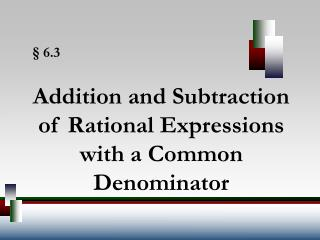DownloadDownload Presentation§ 6.3

# § 6.3

Download Presentation## § 6.3

- - - - - - - - - - - - - - - - - - - - - - - - - - - E N D - - - - - - - - - - - - - - - - - - - - - - - - - - -
##### Presentation Transcript

1. Addition and Subtraction of Rational Expressions with a Common Denominator § 6.3

2. 5 5 2 2 Add . + + 12 12 12 12 7 = 12 Adding/Subtracting Fractions

3. Subtract Common Denominators • Add or subtract the numerators. • Place the sum or difference of the numerators found in step 1 over the common denominator. • Simplify the fraction if possible.

4. a.) Add Common Denominators Example:

5. b.) Subtract Common Denominators Example:

6. Least Common Denominator • Factor each denominator completely. Any factors used more than once should be expressed as powers. • List all different factors that appear in any of the denominators. When the same factor appears in more than one denominator, write that factor with the highest power that appears. • The least common denominator (LCD) is the product of all the factors listed in step 2.

7. a.) b.) c.) Least Common Denominator Find the LCD: The LCD is (2x + 5)(x – 5). The LCD is x(x + 1). The LCD is 36w5z4.